+关注继续查看

# 《python机器学习从入门到高级》分类算法：（上）

• ✨本文收录于《python机器学习从入门到高级》专栏，此专栏主要记录如何使用python实现机器学习模型，尽量坚持每周持续更新，欢迎大家订阅！
• 🌸个人主页：JoJo的数据分析历险记
• 📝个人介绍：小编大四统计在读，目前保研到统计学top3高校继续攻读统计研究生
• 💌如果文章对你有帮助，欢迎✌关注、👍点赞、✌收藏、👍订阅专栏

@TOC

# 导入相关库
import sklearn
import pandas as pd
import numpy as np
import matplotlib.pyplot as plt

# 🌳1. 数据准备

# 导入mnist数据集
from sklearn.datasets import fetch_openml
mnist = fetch_openml('mnist_784', version=1, as_frame=False)
mnist.keys()
dict_keys(['data', 'target', 'frame', 'categories', 'feature_names', 'target_names', 'DESCR', 'details', 'url'])



X, y = mnist["data"], mnist["target"]
X.shape,y.shape
((70000, 784), (70000,))



%matplotlib inline
import matplotlib as mpl
digit = X
digit_image = digit.reshape((28, 28))#还原成28×28
plt.imshow(digit_image, cmap=mpl.cm.binary)
plt.axis("off")
plt.savefig("some_digit_plot")
plt.show()y
'5'



y = y.astype(np.uint8)#将y转换成整数
X_train, X_test, y_train, y_test = X[:60000], X[60000:], y[:60000], y[60000:]

# 🌴2.简单二元分类实现

y_train_7 = (y_train == 7)
y_test_7 = (y_test == 7)

from sklearn.linear_model import SGDClassifier
sgd_clf = SGDClassifier(max_iter=1000, tol=1e-3, random_state=123)#设置random_state为了结果的重复性
sgd_clf.fit(X_train, y_train_7)
SGDClassifier(random_state=123)



sgd_clf.predict(X.reshape((1,-1)))
array([False])



# 🌵3.模型评估

## 🌾3.1 准确率

from sklearn.model_selection import cross_val_score
cross_val_score(sgd_clf, X_train, y_train_7, cv=3, scoring="accuracy")
array([0.97565, 0.97655, 0.963  ])



## 🌿3.2 混淆矩阵

y_train_pred = sgd_clf.predict(X_train)
from sklearn.metrics import confusion_matrix
confusion_matrix(y_train_7, y_train_pred)
array([[53304,   431],
[  550,  5715]], dtype=int64)



## ☘️3.3 召回率和精确度

from sklearn.metrics import precision_score, recall_score

print('precision:',precision_score(y_train_7, y_train_pred))
print('recall:',recall_score(y_train_7,y_train_pred))
precision: 0.929873088187439
recall: 0.9122106943335994



## 🍁3.4 ROC曲线

from sklearn.metrics import roc_curve

fpr, tpr, thresholds = roc_curve(y_train_7, y_scores)
plt.plot(fpr, tpr, linewidth=2)
plt.plot([0, 1], [0, 1], 'k--')
plt.axis([0, 1, 0, 1])
plt.xlabel('False Positive Rate (Fall-Out)', fontsize=16)
plt.ylabel('True Positive Rate (Recall)', fontsize=16)
plt.grid(True)# 🎄推荐文章Python机器学习

7 0C++与Python：哪种语言更适合机器学习

19 0Python机器学习：Scikit-learn
Scikit-learn 是基于 Python 语言的机器学习工具库，它提供了诸如分类、回归、聚类等常用机器学习任务的 API，同时提供了许多常用的数据预处理工具和数据可视化工具。Scikit-learn 的设计旨在与 NumPy、SciPy 和 matplotlib 工具一起使用，因此可以轻松地与这些库进行集成。
13 0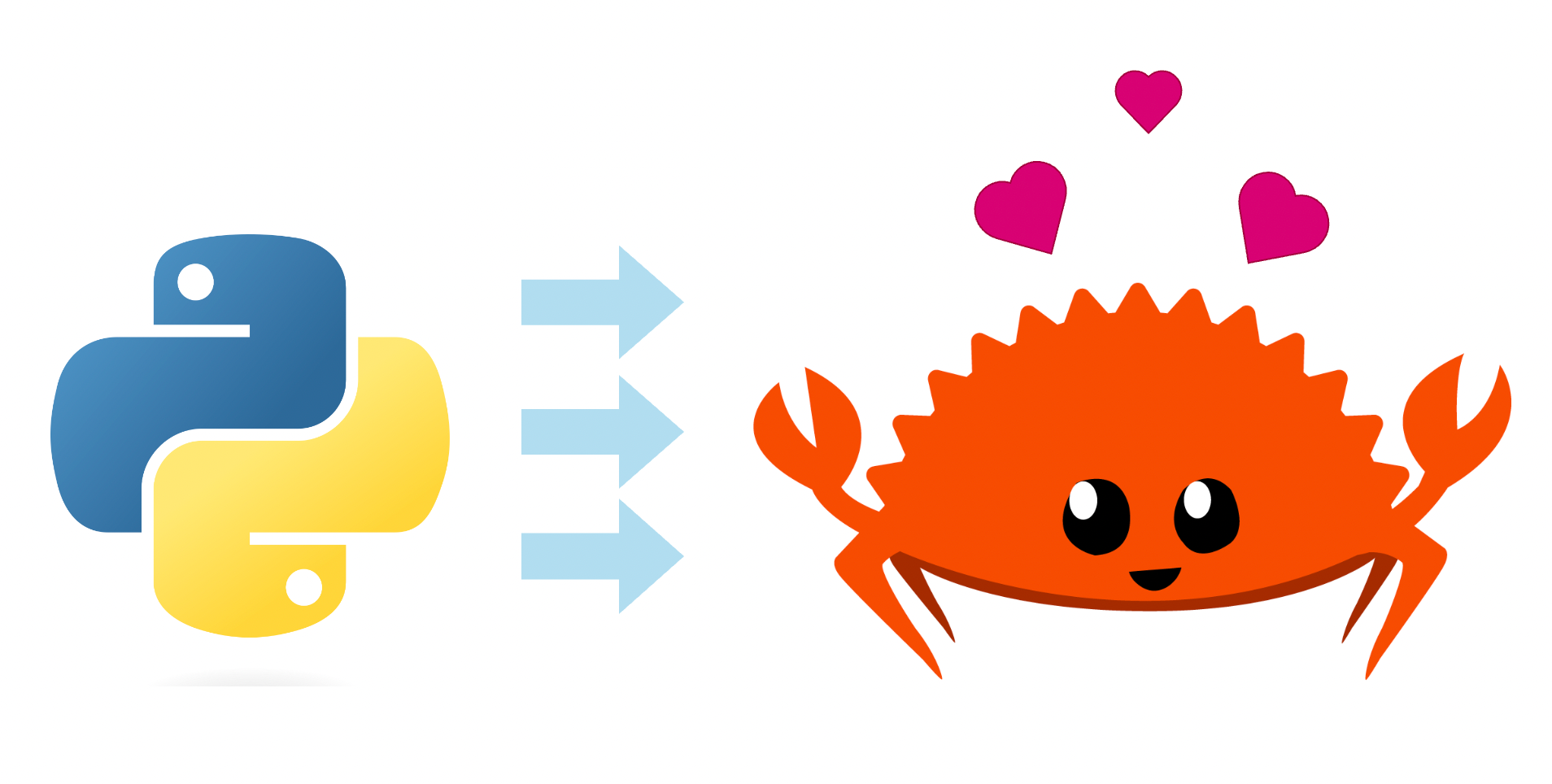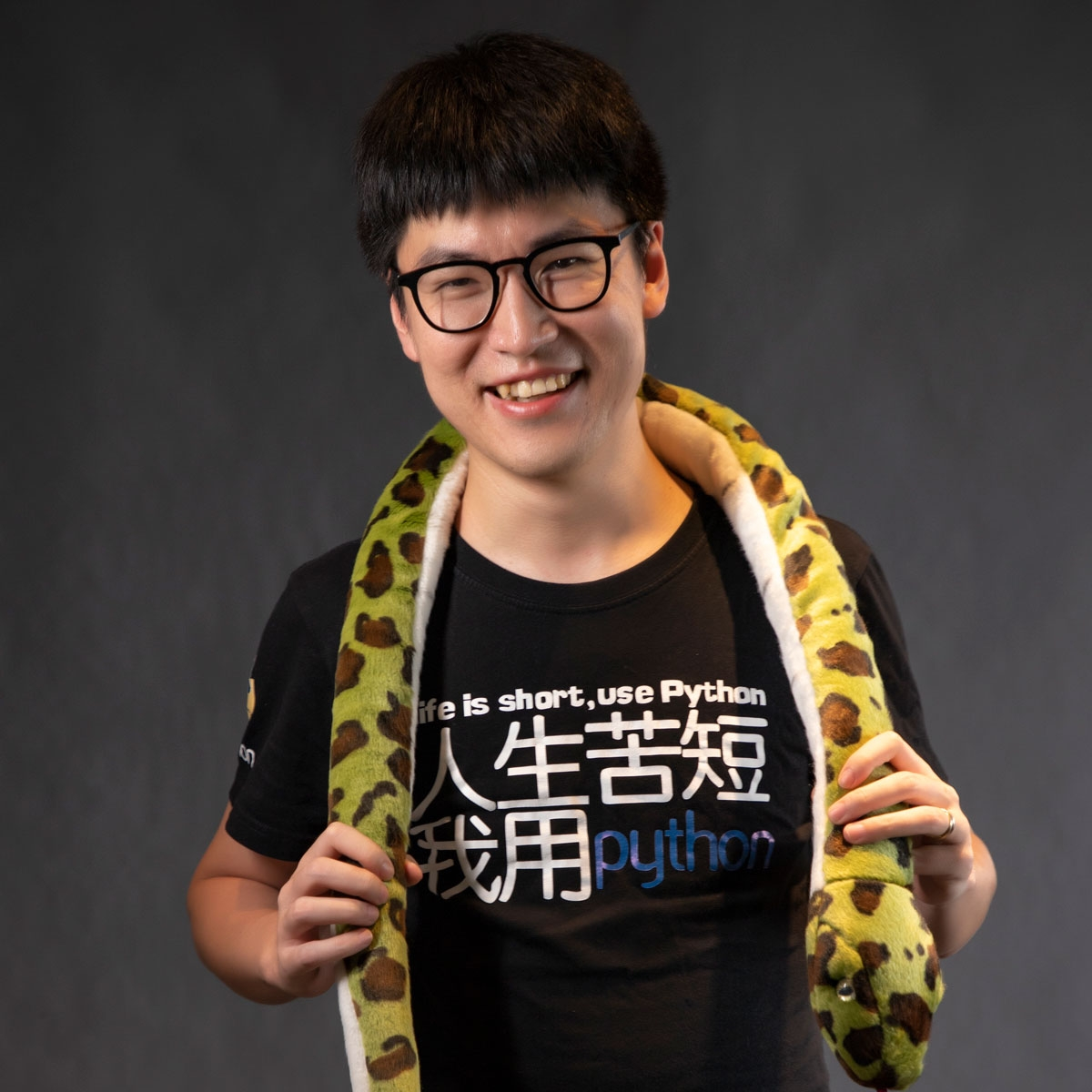Rust语言诞生于2010年，一种多范式、系统级、高级通用编程语言，旨在提高性能和安全性，特别是无畏并发。虽然与Python相比，Rust还年轻，很多库还在开发中，但Rust社区非常活跃并且增长迅猛。很多大厂都是Rust基金会的成员，都在积极地用Rust重构底层基础设施和关键系统应用。
17 0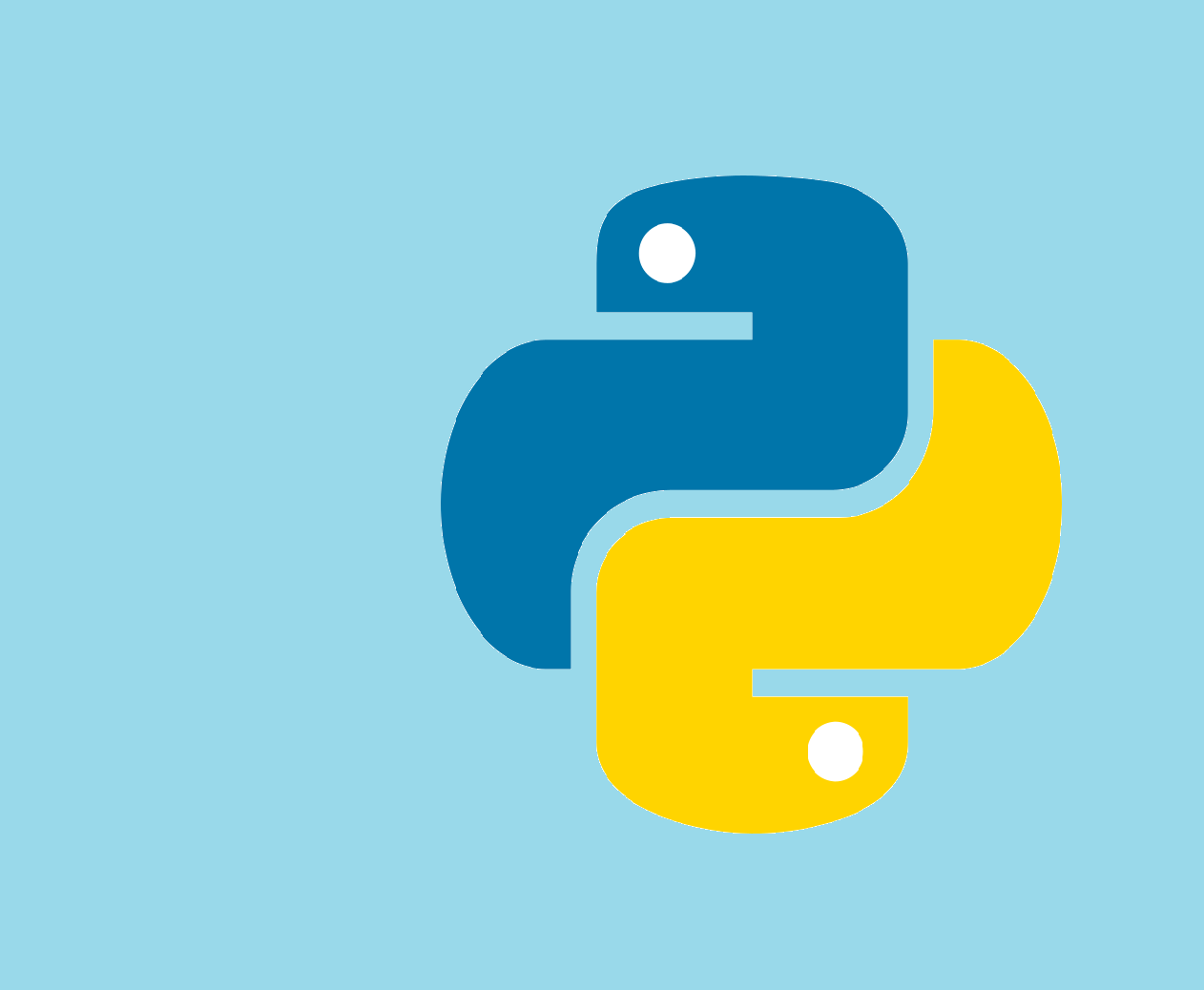14 019 0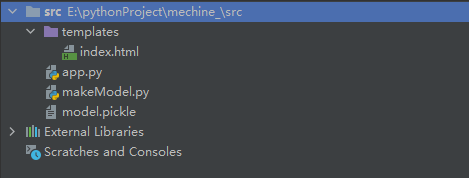【Python】fastapi框架之Web部署机器学习模型
【Python】fastapi框架之Web部署机器学习模型
82 0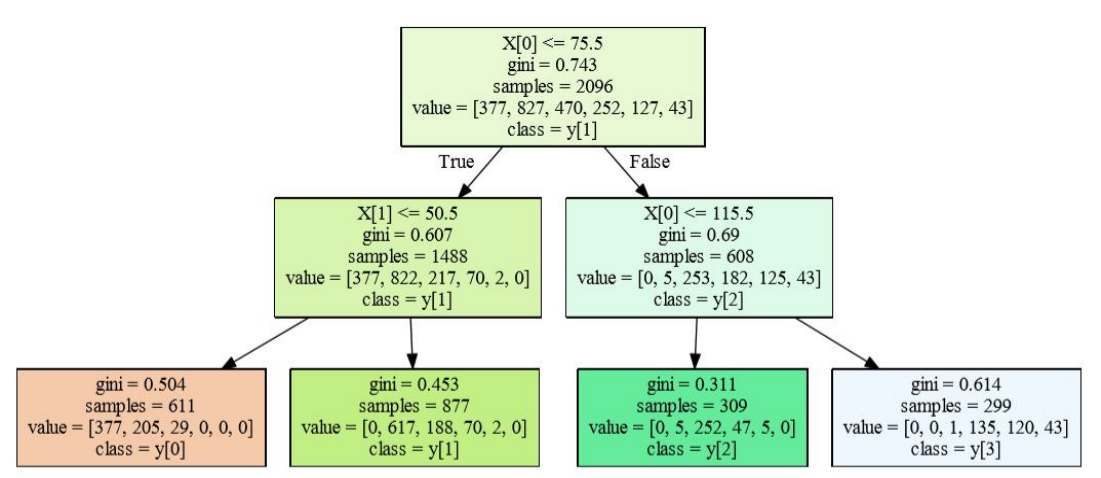python机器学习数据建模与分析——数据预测与预测建模

65 0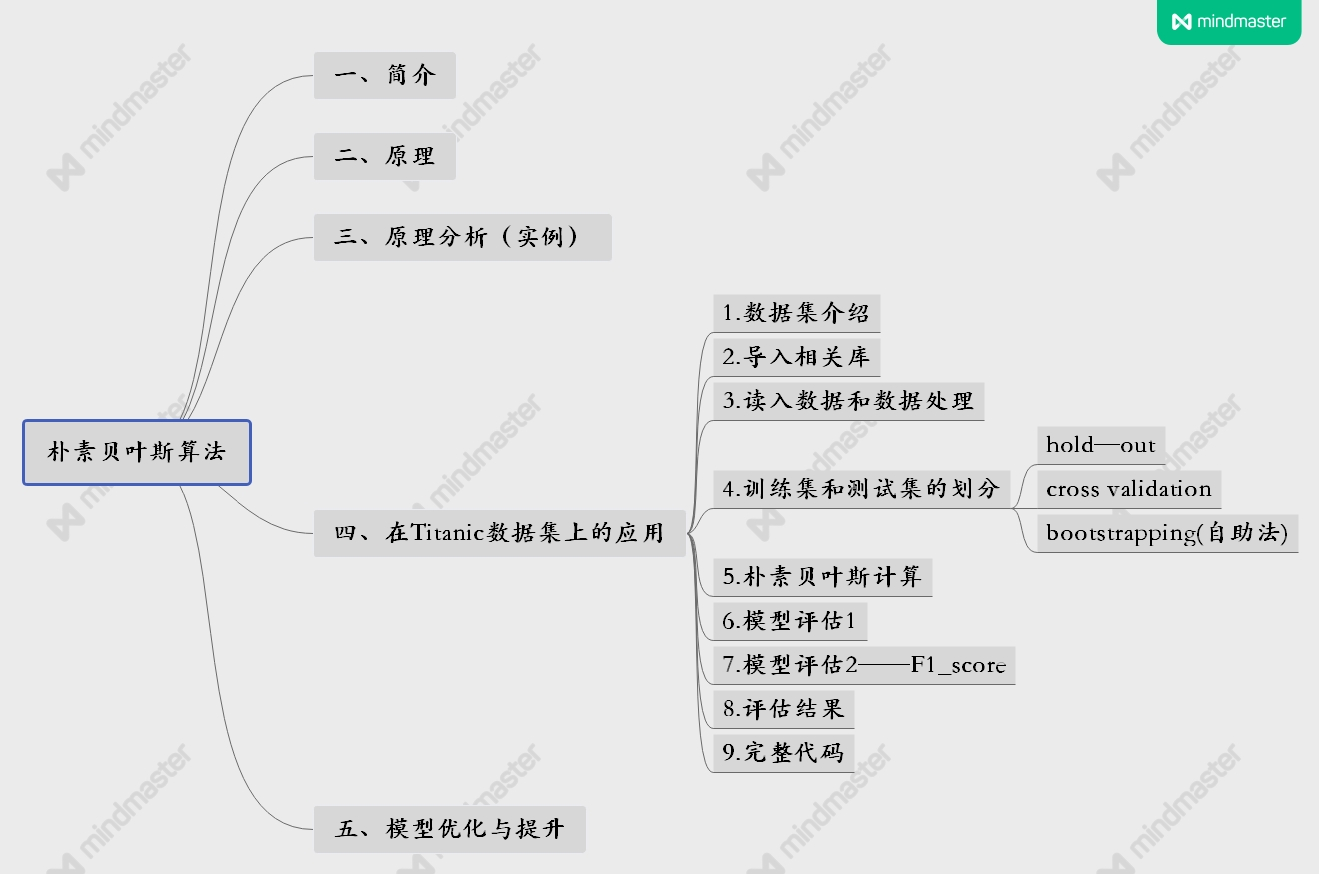python机器学习——朴素贝叶斯算法笔记详细记录

37 0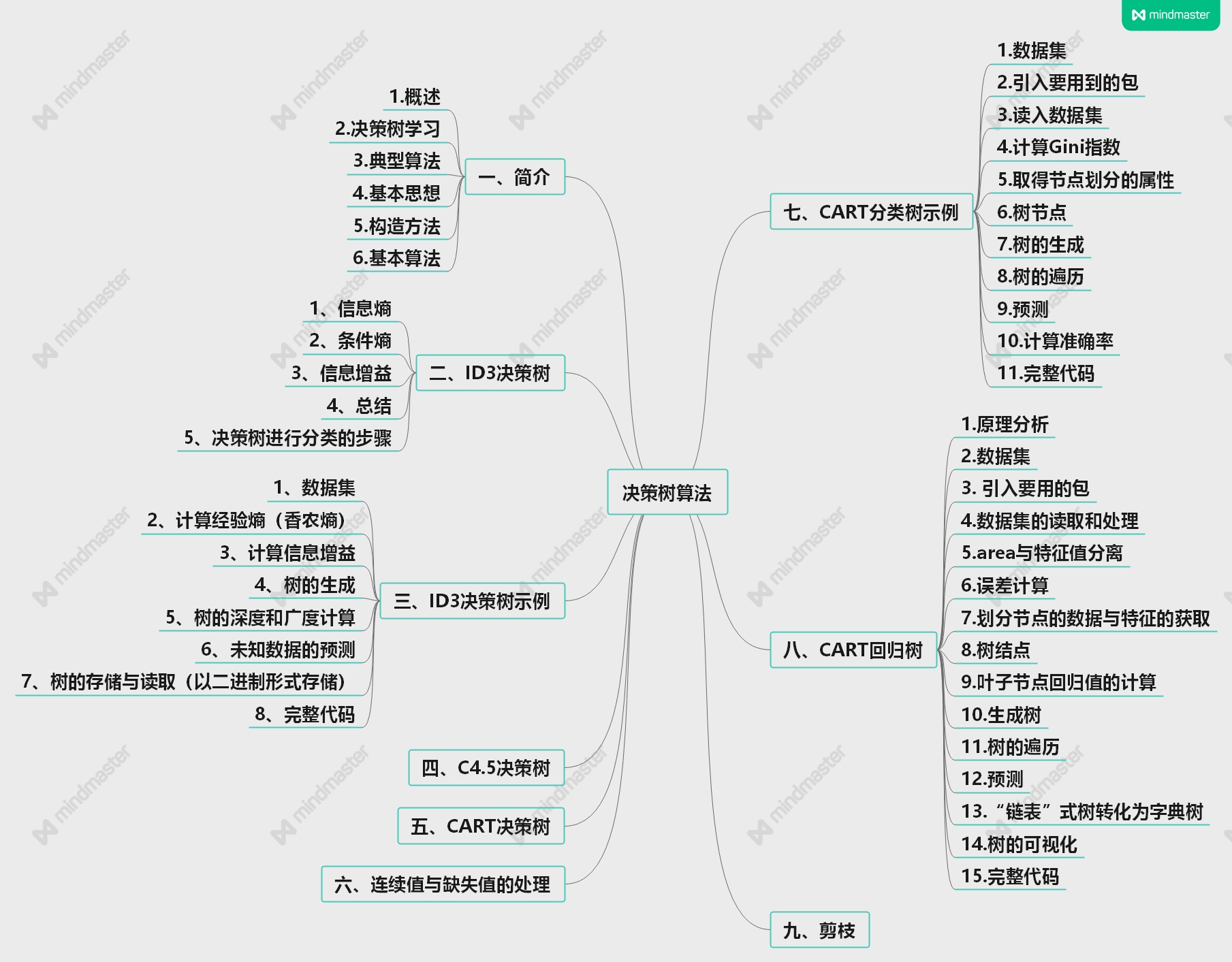python机器学习课程——决策树全网最详解超详细笔记附代码

48 0

【Python入门】Python数据处理编程基础3396

PAI分布式机器学习平台